# Package MKmisc

## Introduction

Package MKmisc includes a collection of functions that I found useful in my daily work. It contains several functions for statistical data analysis; e.g. for sample size and power calculations, computation of confidence intervals, and generation of similarity matrices.

library(MKmisc)

## Descriptive Statistics

### IQR

I implemented function IQrange before the standard function IQR gained the type argument. Since 2010 (r53643, r53644) the function is identical to function IQR.

x <- rnorm(100)
IQrange(x)
##  1.214648
IQR(x)
##  1.214648

It is also possible to compute a standardized version of the IQR leading to a normal-consistent estimate of the standard deviation.

sIQR(x)
##  0.9004196
sd(x)
##  0.9467541

### Mean Absolute Deviation

The mean absolute deviation under the assumption of symmetry is a robust alternative to the sample standard deviation.

meanAD(x)
##  0.9198986

### Five Number Summary

There is a function that computes a so-called five number summary which in contrast to function fivenum uses the first and third quartile instead of the lower and upper hinge.

fiveNS(x)
##     Minimum         25%      Median         75%     Maximum
## -2.57330400 -0.69482207 -0.08864456  0.51982554  2.24396794

### Coefficient of Variation (CV)

There are functions to compute the (classical) coefficient of variation as well as two robust variants. In case of the robust variants, the mean is replaced by the median and the SD is replaced by the (standardized) MAD and the (standardized) IQR, respectively.

## 5% outliers
out <- rbinom(100, prob = 0.05, size = 1)
sum(out)
##  7
x <- (1-out)*rnorm(100, mean = 10, sd = 2) + out*25
CV(x)
##  0.3879403
medCV(x)
##  0.1683868
iqrCV(x)
##  0.1562934

### Signal to Noise Ratio (SNR)

There are functions to compute the (classical) signal to noise ratio as well as two robust variants. In case of the robust variants, the mean is replaced by the median and the SD is replaced by the (standardized) MAD and the (standardized) IQR, respectively.

SNR(x)
##  2.577716
medSNR(x)
##  5.938708
iqrSNR(x)
##  6.398224

### Box- and Whisker-Plot

In contrast to the standard function boxplot which uses the lower and upper hinge for defining the box and the whiskers, the function qboxplot uses the first and third quartile.

x <- rt(10, df = 3)
par(mfrow = c(1,2))
qboxplot(x, main = "1st and 3rd quartile")
boxplot(x, main = "Lower and upper hinge")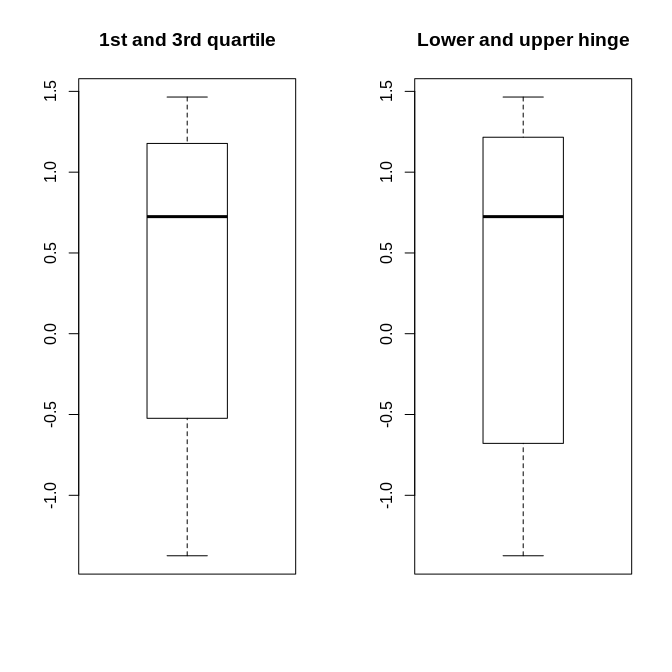The difference between the two versions often is hardly visible.

### OR, RR and Other Risk Measures

Given the incidence of the outcome of interest in the nonexposed (p0) and exposed (p1) group, several risk measures can be computed.

## Example from Wikipedia
risks(p0 = 0.4, p1 = 0.1)
##        p0        p1        RR        OR       RRR       ARR       NNT
## 0.4000000 0.1000000 0.2500000 0.1666667 0.7500000 0.3000000 3.3333333
risks(p0 = 0.4, p1 = 0.5)
##    p0    p1    RR    OR   RRI   ARI   NNH
##  0.40  0.50  1.25  1.50  0.25  0.10 10.00

Given p0 or p1 and OR, we can compute the respective RR.

or2rr(or = 1.5, p0 = 0.4)
##  1.25
or2rr(or = 1/6, p1 = 0.1)
##  0.25

### Generalized Logarithm

The generalized logarithm may be useful as a variance stabilizing transformation when also negative values are present.

curve(log, from = -3, to = 5)
## Warning in log(x): NaNs wurden erzeugt
curve(glog, from = -3, to = 5, add = TRUE, col = "orange")
legend("topleft", fill = c("black", "orange"), legend = c("log", "glog"))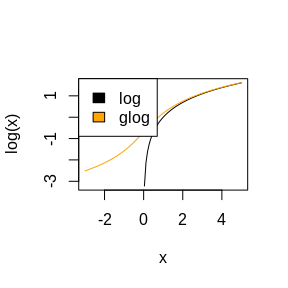As in case of function log there is also glog10 and glog2.

curve(log10(x), from = -3, to = 5)
## Warning in eval(expr, envir = ll, enclos = parent.frame()): NaNs wurden
## erzeugt
curve(glog10(x), from = -3, to = 5, add = TRUE, col = "orange")
legend("topleft", fill = c("black", "orange"), legend = c("log10", "glog10"))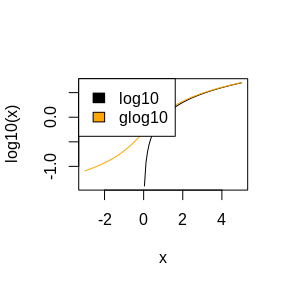There are also functions that compute the inverse of the generalized logarithm.

inv.glog(glog(10))
##  10
inv.glog(glog(10, base = 3), base = 3)
##  10
inv.glog10(glog10(10))
##  10
inv.glog2(glog2(10))
##  10

### Simulate Correlated Variables

To demonstrate Pearson correlation in my lectures, I have written this simple function to simulate correlated variables and to generate a scatter plot of the data.

res <- simCorVars(n = 500, r = 0.8)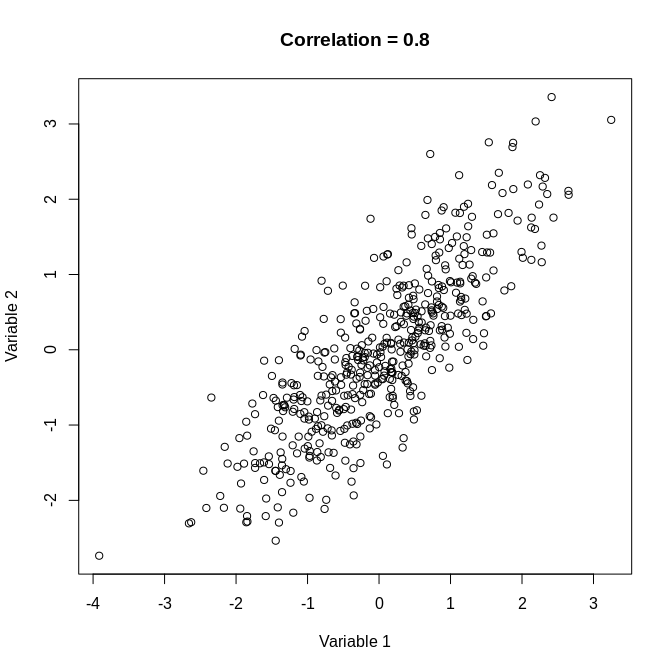cor(res$Var1, res$Var2)
##  0.8222099

### Plot TSH, fT3 and fT4 Values

The thyroid function is usually investigated by determining the values of TSH, fT3 and fT4. The function thyroid can be used to visualize the measured values as relative values with respect to the provided reference ranges.

thyroid(TSH = 1.5, fT3 = 2.5, fT4 = 14, TSHref = c(0.2, 3.0),
fT3ref = c(1.7, 4.2), fT4ref = c(7.6, 15.0))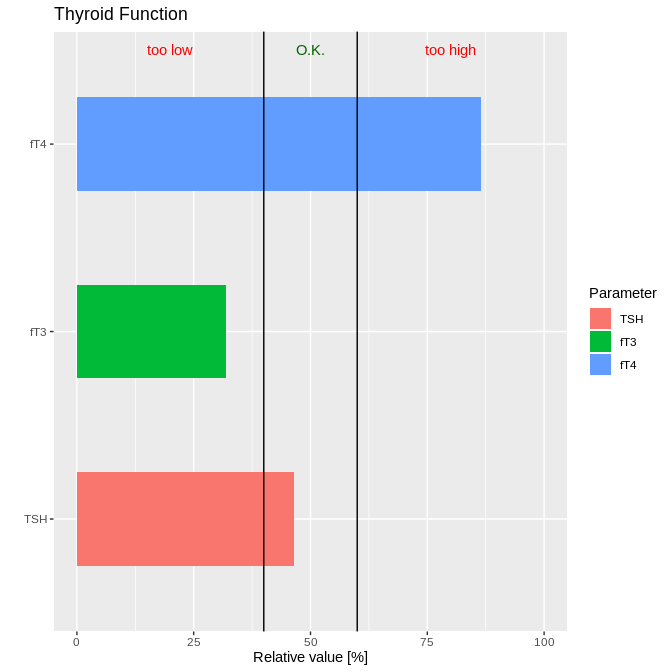### Generalized and Negative Logarithm as Transformations

We can use the generalized logarithm for transforming the axes in ggplot2 plots.

library(ggplot2)
data(mpg)
p1 <- ggplot(mpg, aes(displ, hwy)) + geom_point()
p1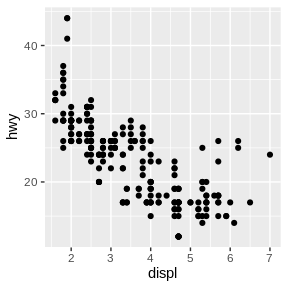p1 + scale_x_log10()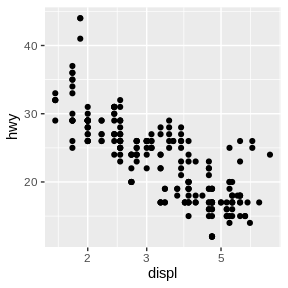p1 + scale_x_glog10()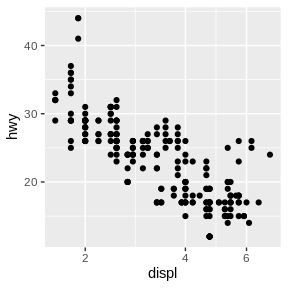p1 + scale_y_log10()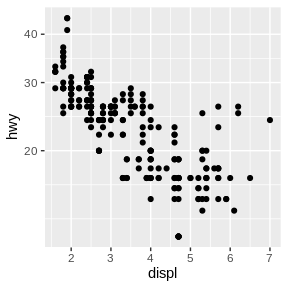p1 + scale_y_glog10()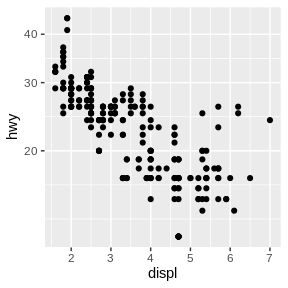The negative logrithm is for instance useful for displaying p values. The interesting values are on the top. This is for instance used in a so-called volcano plot.

x <- matrix(rnorm(1000, mean = 10), nrow = 10)
g1 <- rep("control", 10)
y1 <- matrix(rnorm(500, mean = 11.25), nrow = 10)
y2 <- matrix(rnorm(500, mean = 9.75), nrow = 10)
g2 <- rep("treatment", 10)
group <- factor(c(g1, g2))
Data <- rbind(x, cbind(y1, y2))
pvals <- apply(Data, 2, function(x, group) t.test(x ~ group)$p.value, group = group) ## compute log-fold change logfc <- function(x, group){ res <- tapply(x, group, mean) log2(res/res) } lfcs <- apply(Data, 2, logfc, group = group) ps <- data.frame(pvals = pvals, logfc = lfcs) ggplot(ps, aes(x = logfc, y = pvals)) + geom_point() + geom_hline(yintercept = 0.05) + scale_y_neglog10() + geom_vline(xintercept = c(-0.1, 0.1)) + xlab("log-fold change") + ylab("-log10(p value)") + ggtitle("A Volcano Plot")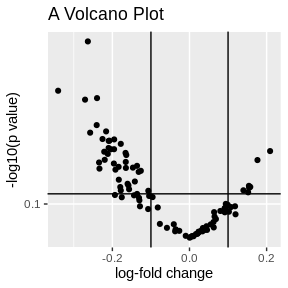### Change Data from Wide to Long Often it’s better to have the data in a long format than in a wide format; e.g., when plotting with package ggplot2. The necessary transformation can be done with function melt.long. library(ggplot2) ## some random data test <- data.frame(x = rnorm(10), y = rnorm(10), z = rnorm(10)) test.long <- melt.long(test) test.long ## value variable ## x1 -1.61097019 x ## x2 -0.17741403 x ## x3 -0.50071863 x ## x4 -0.62530413 x ## x5 -0.83880633 x ## x6 0.73692910 x ## x7 -1.12017453 x ## x8 0.25378171 x ## x9 -0.79050214 x ## x10 -0.90549324 x ## y1 0.37064866 y ## y2 -0.34972516 y ## y3 -0.01794129 y ## y4 0.42985343 y ## y5 1.01118395 y ## y6 0.78036989 y ## y7 0.40400337 y ## y8 0.52458138 y ## y9 0.48055958 y ## y10 0.15980025 y ## z1 0.14791703 z ## z2 0.97722176 z ## z3 0.17527069 z ## z4 -1.64406119 z ## z5 1.53996956 z ## z6 -1.38681726 z ## z7 -0.85355752 z ## z8 0.71800536 z ## z9 0.59257651 z ## z10 -0.45050563 z ggplot(test.long, aes(x = variable, y = value)) + geom_boxplot(aes(fill = variable))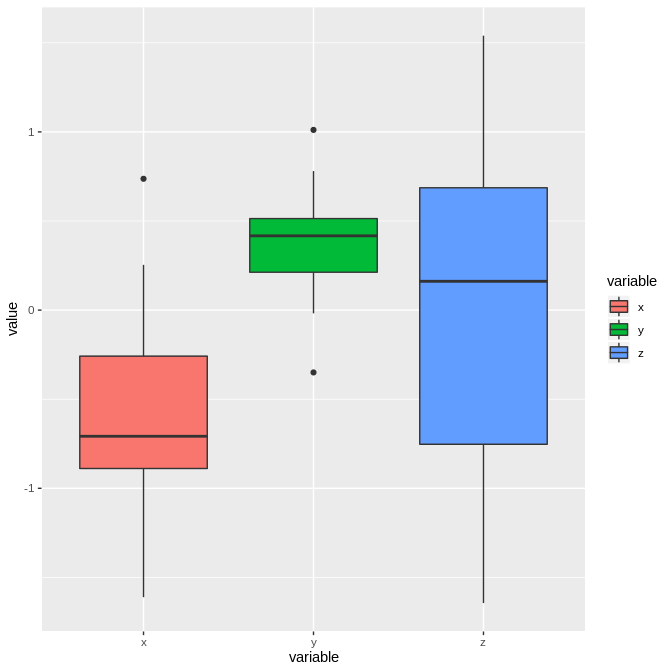## introducing an additional grouping variable group <- factor(rep(c("a","b"), each = 5)) test.long.gr <- melt.long(test, select = 1:2, group = group) test.long.gr ## group value variable ## x1 a -1.61097019 x ## x2 a -0.17741403 x ## x3 a -0.50071863 x ## x4 a -0.62530413 x ## x5 a -0.83880633 x ## x6 b 0.73692910 x ## x7 b -1.12017453 x ## x8 b 0.25378171 x ## x9 b -0.79050214 x ## x10 b -0.90549324 x ## y1 a 0.37064866 y ## y2 a -0.34972516 y ## y3 a -0.01794129 y ## y4 a 0.42985343 y ## y5 a 1.01118395 y ## y6 b 0.78036989 y ## y7 b 0.40400337 y ## y8 b 0.52458138 y ## y9 b 0.48055958 y ## y10 b 0.15980025 y ggplot(test.long.gr, aes(x = variable, y = value, fill = group)) + geom_boxplot()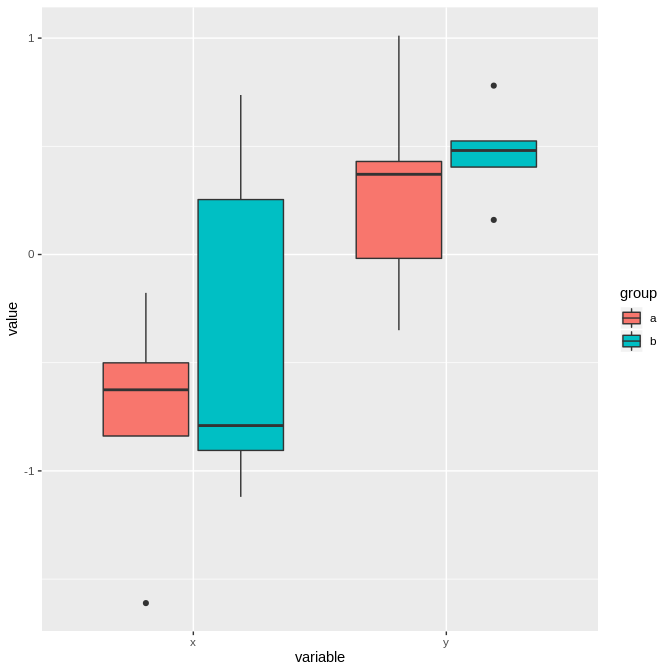## Confidence Intervals ### Binomial Proportion There are several functions for computing confidence intervals. We can compute 10 different confidence intervals for binomial proportions; e.g. ## default: "wilson" binomCI(x = 12, n = 50) ## ## wilson confidence interval ## ## 95 percent confidence interval: ## 2.5 % 97.5 % ## prob 0.1429739 0.3741268 ## ## sample estimate: ## prob ## 0.24 ## ## additional information: ## standard error of prob ## 0.05896867 ## Clopper-Pearson interval binomCI(x = 12, n = 50, method = "clopper-pearson") ## ## clopper-pearson confidence interval ## ## 95 percent confidence interval: ## 2.5 % 97.5 % ## prob 0.1306099 0.3816907 ## ## sample estimate: ## prob ## 0.24 ## identical to binom.test(x = 12, n = 50)$conf.int
##  0.1306099 0.3816907
## attr(,"conf.level")
##  0.95

For all intervals implemented see the help page of function binomCI.

### Mean and SD

We can compute confidence intervals for mean and SD of a normal distribution.

x <- rnorm(50, mean = 2, sd = 3)
## mean and SD unknown
normCI(x)
##
##  Exact confidence interval(s)
##
## 95 percent confidence intervals:
##         2.5 %   97.5 %
## mean 2.225350 4.237621
## sd   2.957314 4.411657
##
## sample estimates:
##     mean       sd
## 3.231486 3.540277
##
## SE of mean
##  0.5006708
## SD known
normCI(x, sd = 3)
##
##  Exact confidence interval(s)
##
## 95 percent confidence interval:
##         2.5 %   97.5 %
## mean 2.399943 4.063028
##
## sample estimate:
##     mean
## 3.231486
##
## SE of mean
##  0.4242641
## mean known
normCI(x, mean = 2)
##
##  Exact confidence interval(s)
##
## 95 percent confidence interval:
##       2.5 %   97.5 %
## sd 2.957314 4.411657
##
## sample estimate:
##       sd
## 3.540277

### Difference in Means

We can compute confidence interval for the difference of means assuming normal distributions.

x <- rnorm(20)
y <- rnorm(20, sd = 2)
## paired
normDiffCI(x, y, paired = TRUE)
##
##  Confidence interval (paired)
##
## 95 percent confidence interval:
##                          2.5 %   97.5 %
## mean of differences -0.0270875 1.399005
##
## sample estimate:
## mean of differences
##            0.685959
##
## SE of mean of differences
##                 0.3406776
## compare
normCI(x-y)
##
##  Exact confidence interval(s)
##
## 95 percent confidence intervals:
##           2.5 %   97.5 %
## mean -0.0270875 1.399005
## sd    1.1586502 2.225264
##
## sample estimates:
##     mean       sd
## 0.685959 1.523557
##
## SE of mean
##  0.3406776
## unpaired
y <- rnorm(10, mean = 1, sd = 2)
## classical
normDiffCI(x, y, method = "classical")
##
##  Classical confidence interval (unpaired)
##
## 95 percent confidence interval:
##                        2.5 %   97.5 %
## difference in means -1.48845 1.163204
##
## sample estimate:
## difference in means
##          -0.1626228
##
## SE of difference in means           Cohen's d (SMD)
##                0.64724785               -0.09730977
##
##   mean of x     SD of x   mean of y     SD of y
## -0.06592175  0.52331863  0.09670105  2.84793963
## Welch (default as in case of function t.test)
normDiffCI(x, y, method = "welch")
##
##  Welch confidence interval (unpaired)
##
## 95 percent confidence interval:
##                         2.5 %  97.5 %
## difference in means -2.206816 1.88157
##
## sample estimate:
## difference in means
##          -0.1626228
##
## SE of difference in means           Cohen's d (SMD)
##                0.90816801               -0.05616163
##
##   mean of x     SD of x   mean of y     SD of y
## -0.06592175  0.52331863  0.09670105  2.84793963
## Hsu
normDiffCI(x, y, method = "hsu")
##
##  Hsu confidence interval (unpaired)
##
## 95 percent confidence interval:
##                         2.5 %   97.5 %
## difference in means -2.217042 1.891796
##
## sample estimate:
## difference in means
##          -0.1626228
##
## SE of difference in means           Cohen's d (SMD)
##                0.90816801               -0.05616163
##
##   mean of x     SD of x   mean of y     SD of y
## -0.06592175  0.52331863  0.09670105  2.84793963

In case of unequal variances and unequal sample sizes per group the classical confidence interval may have a bad coverage (too long or too short), as is indicated by the small Monte-Carlo simulation study below.

M <- 100
CIhsu <- CIwelch <- CIclass <- matrix(NA, nrow = M, ncol = 2)
for(i in 1:M){
x <- rnorm(10)
y <- rnorm(30, sd = 0.1)
CIclass[i,] <- normDiffCI(x, y, method = "classical")$conf.int CIwelch[i,] <- normDiffCI(x, y, method = "welch")$conf.int
CIhsu[i,] <- normDiffCI(x, y, method = "hsu")$conf.int } ## coverage probabilies ## classical sum(CIclass[,1] < 0 & 0 < CIclass[,2])/M ##  0.74 ## Welch sum(CIwelch[,1] < 0 & 0 < CIwelch[,2])/M ##  0.96 ## Hsu sum(CIhsu[,1] < 0 & 0 < CIhsu[,2])/M ##  0.96 ### Coefficient of Variation We provide 11 different confidence intervals for the (classical) coefficient of variation; e.g. x <- rnorm(100, mean = 10, sd = 2) # CV = 0.2 ## default: "miller" cvCI(x) ## ## Miller (1991) confidence interval ## ## 95 percent confidence interval: ## 2.5 % 97.5 % ## CV 0.1680721 0.2248794 ## ## sample estimate: ## CV ## 0.1964758 ## ## additional information: ## standard error of CV ## 0.01449191 ## Gulhar et al. (2012) cvCI(x, method = "gulhar") ## ## Gulhar et al (2012) confidence interval ## ## 95 percent confidence interval: ## 2.5 % 97.5 % ## CV 0.1725071 0.228241 ## ## sample estimate: ## CV ## 0.1964758 For all intervals implemented see the help page of function cvCI. ### Quantiles, Median and MAD We start with the computation of confidence intervals for quantiles. x <- rexp(100, rate = 0.5) ## exact quantileCI(x = x, prob = 0.95) ## ## exact confidence interval ## ## 95.14464 percent confidence interval: ## lower upper ## 95 % quantile 5.536315 9.984989 ## ## sample estimate: ## 95 % quantile ## 6.546731 ## asymptotic quantileCI(x = x, prob = 0.95, method = "asymptotic") ## ## asymptotic confidence interval ## ## 95 percent confidence interval: ## 2.5 % 97.5 % ## 95 % quantile 5.536315 10.56759 ## ## sample estimate: ## 95 % quantile ## 6.546731 Next, we consider the median. ## exact medianCI(x = x) ## ## exact confidence interval ## ## 95.23684 percent confidence intervals: ## lower upper ## median 1.145691 2.171415 ## median 1.287856 2.371827 ## ## sample estimate: ## median ## 1.682672 ## asymptotic medianCI(x = x, method = "asymptotic") ## ## asymptotic confidence interval ## ## 95 percent confidence interval: ## 2.5 % 97.5 % ## median 1.188723 2.194297 ## ## sample estimate: ## median ## 1.682672 It often happens that quantile confidence intervals are not unique. Here the minimum length interval might be of interest. medianCI(x = x, minLength = TRUE) ## ## minimum length exact confidence interval ## ## 95.23684 percent confidence interval: ## lower upper ## median 1.145691 2.171415 ## ## sample estimate: ## median ## 1.682672 Finally, we take a look at MAD (median absolute deviation) where by default the standardized MAD is used (see function mad). ## exact madCI(x = x) ## ## exact confidence interval ## ## 95.23684 percent confidence intervals: ## lower upper ## MAD 1.283361 2.199345 ## MAD 1.431107 2.224908 ## ## sample estimate: ## MAD ## 1.845505 ## aysymptotic madCI(x = x, method = "asymptotic") ## ## asymptotic confidence interval ## ## 95 percent confidence interval: ## 2.5 % 97.5 % ## MAD 1.39216 2.201539 ## ## sample estimate: ## MAD ## 1.845505 ## unstandardized madCI(x = x, constant = 1) ## ## exact confidence interval ## ## 95.23684 percent confidence intervals: ## lower upper ## MAD 0.8656152 1.483438 ## MAD 0.9652688 1.500680 ## ## sample estimate: ## MAD ## 1.244776 ### Relative Risk There is also a function for computing an approximate confidence interval for the relative risk (RR). ## Example from Wikipedia rrCI(a = 15, b = 135, c = 100, d = 150) ## ## asymptotic confidence interval ## ## 95 percent confidence interval: ## 2.5 % 97.5 % ## relative risk 0.1510993 0.4136353 ## ## sample estimate: ## relative risk ## 0.25 rrCI(a = 75, b = 75, c = 100, d = 150) ## ## asymptotic confidence interval ## ## 95 percent confidence interval: ## 2.5 % 97.5 % ## relative risk 1.00256 1.55851 ## ## sample estimate: ## relative risk ## 1.25 ## Sample Size ### Welch Two-Sample t-Test For computing the sample size of the Welch t-test, we only consider the situation of equal group size (balanced design). ## identical results as power.t.test, since sd = sd1 = sd2 = 1 power.welch.t.test(n = 20, delta = 1) ## ## Two-sample Welch t test power calculation ## ## n = 20 ## delta = 1 ## sd1 = 1 ## sd2 = 1 ## sig.level = 0.05 ## power = 0.8689528 ## alternative = two.sided ## ## NOTE: n is number in *each* group power.welch.t.test(power = .90, delta = 1) ## ## Two-sample Welch t test power calculation ## ## n = 22.0211 ## delta = 1 ## sd1 = 1 ## sd2 = 1 ## sig.level = 0.05 ## power = 0.9 ## alternative = two.sided ## ## NOTE: n is number in *each* group power.welch.t.test(power = .90, delta = 1, alternative = "one.sided") ## ## Two-sample Welch t test power calculation ## ## n = 17.84713 ## delta = 1 ## sd1 = 1 ## sd2 = 1 ## sig.level = 0.05 ## power = 0.9 ## alternative = one.sided ## ## NOTE: n is number in *each* group ## sd1 = 0.5, sd2 = 1 power.welch.t.test(delta = 1, sd1 = 0.5, sd2 = 1, power = 0.9) ## ## Two-sample Welch t test power calculation ## ## n = 14.53583 ## delta = 1 ## sd1 = 0.5 ## sd2 = 1 ## sig.level = 0.05 ## power = 0.9 ## alternative = two.sided ## ## NOTE: n is number in *each* group ### Hsu Two-Sample t-Test For computing the sample size of the Hsu t-test, we only consider the situation of equal group size (balanced design). ## slightly more conservative than Welch t-test power.hsu.t.test(n = 20, delta = 1) ## ## Two-sample Hsu t test power calculation ## ## n = 20 ## delta = 1 ## sd1 = 1 ## sd2 = 1 ## sig.level = 0.05 ## power = 0.8506046 ## alternative = two.sided ## ## NOTE: n is number in *each* group power.hsu.t.test(power = .90, delta = 1) ## ## Two-sample Hsu t test power calculation ## ## n = 23.02186 ## delta = 1 ## sd1 = 1 ## sd2 = 1 ## sig.level = 0.05 ## power = 0.9 ## alternative = two.sided ## ## NOTE: n is number in *each* group power.hsu.t.test(power = .90, delta = 1, alternative = "one.sided") ## ## Two-sample Hsu t test power calculation ## ## n = 18.5674 ## delta = 1 ## sd1 = 1 ## sd2 = 1 ## sig.level = 0.05 ## power = 0.9 ## alternative = one.sided ## ## NOTE: n is number in *each* group ## sd1 = 0.5, sd2 = 1 power.welch.t.test(delta = 0.5, sd1 = 0.5, sd2 = 1, power = 0.9) ## ## Two-sample Welch t test power calculation ## ## n = 53.86822 ## delta = 0.5 ## sd1 = 0.5 ## sd2 = 1 ## sig.level = 0.05 ## power = 0.9 ## alternative = two.sided ## ## NOTE: n is number in *each* group power.hsu.t.test(delta = 0.5, sd1 = 0.5, sd2 = 1, power = 0.9) ## ## Two-sample Hsu t test power calculation ## ## n = 54.49421 ## delta = 0.5 ## sd1 = 0.5 ## sd2 = 1 ## sig.level = 0.05 ## power = 0.9 ## alternative = two.sided ## ## NOTE: n is number in *each* group ### Two Negative Binomial Rates When we consider two negative binomial rates, we can compute sample size or power applying function power.nb.test. ## examples from Table III in Zhu and Lakkis (2014) power.nb.test(mu0 = 5.0, RR = 2.0, theta = 1/0.5, duration = 1, power = 0.8, approach = 1) ## ## Power calculation for comparing two negative binomial rates ## ## n = 22.37386 ## n1 = 22.37386 ## mu0 = 5 ## RR = 2 ## theta = 2 ## duration = 1 ## sig.level = 0.05 ## power = 0.8 ## alternative = two.sided ## ## NOTE: n = sample size of control group, n1 = sample size of treatment group power.nb.test(mu0 = 5.0, RR = 2.0, theta = 1/0.5, duration = 1, power = 0.8, approach = 2) ## ## Power calculation for comparing two negative binomial rates ## ## n = 21.23734 ## n1 = 21.23734 ## mu0 = 5 ## RR = 2 ## theta = 2 ## duration = 1 ## sig.level = 0.05 ## power = 0.8 ## alternative = two.sided ## ## NOTE: n = sample size of control group, n1 = sample size of treatment group power.nb.test(mu0 = 5.0, RR = 2.0, theta = 1/0.5, duration = 1, power = 0.8, approach = 3) ## ## Power calculation for comparing two negative binomial rates ## ## n = 20.85564 ## n1 = 20.85564 ## mu0 = 5 ## RR = 2 ## theta = 2 ## duration = 1 ## sig.level = 0.05 ## power = 0.8 ## alternative = two.sided ## ## NOTE: n = sample size of control group, n1 = sample size of treatment group ## Moderated Tests Based on Package limma ### Moderated t-Test The function to compute the moderated t-test was motivated by the fact that my students have problems to understand and correctly adapt the code of the limma package. ## One-sample test X <- matrix(rnorm(10*20, mean = 1), nrow = 10, ncol = 20) mod.t.test(X) ## mean 2.5% 97.5% t p.value adj.p.value ## 1 0.5087996 0.1038840 0.9137152 2.513590 1.465866e-02 1.465866e-02 ## 2 0.7813481 0.3622029 1.2004933 3.728998 4.288804e-04 5.361005e-04 ## 3 0.8918729 0.4564595 1.3272863 4.097447 1.274410e-04 1.820585e-04 ## 4 1.1559184 0.7110024 1.6008343 5.197103 2.569496e-06 6.853782e-06 ## 5 1.2209407 0.8060725 1.6358090 5.887034 1.906366e-07 9.531828e-07 ## 6 1.2645726 0.7761898 1.7529555 5.179591 2.741513e-06 6.853782e-06 ## 7 1.4837387 1.0657152 1.9017623 7.100171 1.703756e-09 1.703756e-08 ## 8 0.9248638 0.5004022 1.3493253 4.358645 5.220173e-05 8.700289e-05 ## 9 0.6746272 0.2008917 1.1483626 2.848657 6.009242e-03 6.676936e-03 ## 10 1.0041004 0.6076027 1.4005981 5.065807 4.169611e-06 8.339222e-06 ## B ## 1 -3.9188424 ## 2 -0.6509869 ## 3 0.5026442 ## 4 4.2647451 ## 5 6.7970983 ## 6 4.2018584 ## 7 11.4165844 ## 8 1.3569612 ## 9 -3.1120015 ## 10 3.7952104 ## Two-sample test set.seed(123) X <- rbind(matrix(rnorm(5*20), nrow = 5, ncol = 20), matrix(rnorm(5*20, mean = 1), nrow = 5, ncol = 20)) g2 <- factor(c(rep("group 1", 10), rep("group 2", 10))) mod.t.test(X, group = g2) ## difference in means 2.5% 97.5% t p.value ## 1 -0.30099317 -1.1337943 0.5318079 -0.71317027 0.4766640 ## 2 0.13637650 -0.6964246 0.9691776 0.32312914 0.7469725 ## 3 -0.49260925 -1.3254104 0.3401919 -1.16718354 0.2446801 ## 4 0.15266680 -0.6801343 0.9854679 0.36172723 0.7179801 ## 5 -0.05546448 -0.8882656 0.7773366 -0.13141699 0.8955922 ## 6 -0.03152278 -0.8643239 0.8012783 -0.07468976 0.9405445 ## 7 -0.69311916 -1.5259203 0.1396820 -1.64226977 0.1022800 ## 8 -0.17595066 -1.0087518 0.6568505 -0.41689577 0.6772514 ## 9 -0.54524220 -1.3780433 0.2875589 -1.29189154 0.1980510 ## 10 -0.01770155 -0.8505027 0.8150996 -0.04194188 0.9665915 ## adj.p.value B mean of group 1 mean of group 2 ## 1 0.9665915 -4.608912 0.07083562 0.371828786 ## 2 0.9665915 -4.619655 0.13562592 -0.000750575 ## 3 0.8156005 -4.586223 -0.30436677 0.188242477 ## 4 0.9665915 -4.618953 0.18497579 0.032308986 ## 5 0.9665915 -4.621971 0.08494719 0.140411667 ## 6 0.9665915 -4.622282 1.11828347 1.149806246 ## 7 0.8156005 -4.550748 0.67404426 1.367163425 ## 8 0.9665915 -4.617811 0.53111339 0.707064052 ## 9 0.8156005 -4.578072 0.92210638 1.467348578 ## 10 0.9665915 -4.622384 0.48495033 0.502651884 ## Paired two-sample test mod.t.test(X, group = g2, paired = TRUE) ## mean of differences 2.5% 97.5% t p.value ## 1 -0.30099317 -0.7260027 0.12401634 -1.39744874 0.163998732 ## 2 0.13637650 -0.2886330 0.56138600 0.63316773 0.527427738 ## 3 -0.49260925 -0.9176188 -0.06759974 -2.28708237 0.023354856 ## 4 0.15266680 -0.2723427 0.57767631 0.70880025 0.479365424 ## 5 -0.05546448 -0.4804740 0.36954503 -0.25751005 0.797079211 ## 6 -0.03152278 -0.4565323 0.39348673 -0.14635370 0.883805952 ## 7 -0.69311916 -1.1181287 -0.26810966 -3.21800824 0.001531067 ## 8 -0.17595066 -0.6009602 0.24905885 -0.81690235 0.415064109 ## 9 -0.54524220 -0.9702517 -0.12023269 -2.53144623 0.012215144 ## 10 -0.01770155 -0.4427111 0.40730796 -0.08218462 0.934591222 ## adj.p.value B ## 1 0.40999683 -5.193223 ## 2 0.75346820 -5.932720 ## 3 0.07784952 -3.631339 ## 4 0.75346820 -5.884358 ## 5 0.93459122 -6.092149 ## 6 0.93459122 -6.113540 ## 7 0.01531067 -1.189400 ## 8 0.75346820 -5.805769 ## 9 0.06107572 -3.070283 ## 10 0.93459122 -6.120528 ### Moderated 1-Way ANOVA The function to compute a moderated 1-way ANOVA was motivated by the fact that my students have problems to understand and correctly adapt the code of the limma package. set.seed(123) X <- rbind(matrix(rnorm(5*20), nrow = 5, ncol = 20), matrix(rnorm(5*20, mean = 1), nrow = 5, ncol = 20)) gr <- factor(c(rep("A1", 5), rep("B2", 5), rep("C3", 5), rep("D4", 5))) mod.oneway.test(X, gr) ## A-B A-C A-D B-C B-D C-D ## 1 1.0974330 0.2271669 0.26827976 -0.87026609 -0.8291532 0.04111286 ## 2 0.0769194 0.9622020 -0.61252965 0.88528264 -0.6894491 -1.57473169 ## 3 -0.3105477 -0.7130963 -0.58266984 -0.40254866 -0.2721221 0.13042651 ## 4 -0.5832304 -0.2440290 -0.03386782 0.33920142 0.5493626 0.21016117 ## 5 -0.9579128 -0.4501003 -0.61874146 0.50781250 0.3391713 -0.16864116 ## 6 -0.6013595 -0.5109767 -0.15342834 0.09038278 0.4479311 0.35754833 ## 7 0.3471695 -0.3493145 -0.68975433 -0.69648400 -1.0369239 -0.34043986 ## 8 -0.9277388 -0.5691081 -0.71053196 0.35863064 0.2172068 -0.14142382 ## 9 -0.5998638 -1.5488157 -0.14153255 -0.94895185 0.4583313 1.40728311 ## 10 1.5527067 0.5179562 0.99934740 -1.03475050 -0.5533593 0.48139118 ## grand mean F p.value adj.p.value ## 1 0.22133220 1.4119467 0.23709800 0.5868380 ## 2 0.06743767 2.5678204 0.05255479 0.1751826 ## 3 -0.05806215 0.6094415 0.60879068 0.7253749 ## 4 0.10864239 0.4386138 0.72537489 0.7253749 ## 5 0.11267943 0.9691456 0.40611786 0.5868380 ## 6 1.13404486 0.5002131 0.68212276 0.7253749 ## 7 1.02060384 1.2185746 0.30111975 0.5868380 ## 8 0.61908872 0.9594030 0.41078662 0.5868380 ## 9 1.19472748 2.9871234 0.02980893 0.1751826 ## 10 0.49380111 2.6903326 0.04456696 0.1751826 ### Pairwise moderated t-tests As a optional post-hoc analysis after mod.oneway.test one can use pairwise moderated t-tests. One should carefully think about the adjustment of p values in this context. pairwise.mod.t.test(X, gr) ## A1-B2 A1-B2: t A1-B2: p A1-B2: adj.p A1-B2: B A1-C3 ## 1 1.0974330 1.9176595 0.056935659 0.26739434 -4.150296 0.2271669 ## 2 0.0769194 0.1344093 0.893247840 0.89324784 -5.826707 0.9622020 ## 3 -0.3105477 -0.5426525 0.588124185 0.65347132 -5.700081 -0.7130963 ## 4 -0.5832304 -1.0191395 0.309675276 0.44239325 -5.359162 -0.2440290 ## 5 -0.9579128 -1.6738612 0.096111665 0.26739434 -4.551426 -0.4501003 ## 6 -0.6013595 -1.0508183 0.294926580 0.44239325 -5.329122 -0.5109767 ## 7 0.3471695 0.6066456 0.544945915 0.65347132 -5.666387 -0.3493145 ## 8 -0.9277388 -1.6211350 0.106957735 0.26739434 -4.631016 -0.5691081 ## 9 -0.5998638 -1.0482048 0.296125067 0.44239325 -5.331635 -1.5488157 ## 10 1.5527067 2.7132069 0.007393498 0.07393498 -2.462559 0.5179562 ## A1-C3: t A1-C3: p A1-C3: adj.p A1-C3: B A1-D4 A1-D4: t ## 1 0.3969525 0.691931480 0.69193148 -5.757494 0.26827976 0.46879329 ## 2 1.6813563 0.094644661 0.47322331 -4.535810 -0.61252965 -1.07033714 ## 3 -1.2460679 0.214560903 0.61819096 -5.119000 -0.58266984 -1.01815997 ## 4 -0.4264174 0.670376894 0.69193148 -5.746391 -0.03386782 -0.05918078 ## 5 -0.7865073 0.432733671 0.61819096 -5.546500 -0.61874146 -1.08119168 ## 6 -0.8928830 0.373260796 0.61819096 -5.464740 -0.15342834 -0.26810139 ## 7 -0.6103937 0.542467061 0.67808383 -5.659092 -0.68975433 -1.20527990 ## 8 -0.9944622 0.321499481 0.61819096 -5.376999 -0.71053196 -1.24158683 ## 9 -2.7064076 0.007539705 0.07539705 -2.477381 -0.14153255 -0.24731463 ## 10 0.9050791 0.366784330 0.61819096 -5.454705 0.99934740 1.74626427 ## A1-D4: p A1-D4: adj.p A1-D4: B B2-C3 B2-C3: t B2-C3: p ## 1 0.63985549 0.8944242 -4.606903 -0.87026609 -1.5207070 0.13030713 ## 2 0.28607955 0.5168984 -4.593186 0.88528264 1.5469470 0.12385169 ## 3 0.31013903 0.5168984 -4.594801 -0.40254866 -0.7034154 0.48281925 ## 4 0.95288197 0.9528820 -4.610107 0.33920142 0.5927221 0.55420392 ## 5 0.28123878 0.5168984 -4.592840 0.50781250 0.8873539 0.37622030 ## 6 0.78896661 0.8944242 -4.609094 0.09038278 0.1579353 0.87470693 ## 7 0.22987482 0.5168984 -4.588637 -0.69648400 -1.2170394 0.22538132 ## 8 0.21620608 0.5168984 -4.587321 0.35863064 0.6266728 0.53176683 ## 9 0.80498180 0.8944242 -4.609252 -0.94895185 -1.6582029 0.09923575 ## 10 0.08268426 0.5168984 -4.564982 -1.03475050 -1.8081278 0.07246459 ## B2-C3: adj.p B2-C3: B B2-D4 B2-D4: t B2-D4: p B2-D4: adj.p ## 1 0.3257678 -4.575899 -0.8291532 -1.4488662 0.14933196 0.6213576 ## 2 0.3257678 -4.574706 -0.6894491 -1.2047465 0.23008017 0.6213576 ## 3 0.6157821 -4.602828 -0.2721221 -0.4755075 0.63507382 0.7047841 ## 4 0.6157821 -4.604954 0.5493626 0.9599587 0.33852439 0.6213576 ## 5 0.6157821 -4.598493 0.3391713 0.5926696 0.55423902 0.6927988 ## 6 0.8747069 -4.609789 0.4479311 0.7827169 0.43495030 0.6213576 ## 7 0.4507626 -4.588215 -1.0369239 -1.8119255 0.07187282 0.6213576 ## 8 0.6157821 -4.604340 0.2172068 0.3795482 0.70478410 0.7047841 ## 9 0.3257678 -4.569423 0.4583313 0.8008902 0.42438292 0.6213576 ## 10 0.3257678 -4.561724 -0.5533593 -0.9669426 0.33503202 0.6213576 ## B2-D4: B C3-D4 C3-D4: t C3-D4: p C3-D4: adj.p C3-D4: B ## 1 -4.579059 0.04111286 0.0718408 0.942818328 0.94281833 -5.862037 ## 2 -4.588656 -1.57473169 -2.7516934 0.006613244 0.06613244 -2.377508 ## 3 -4.606809 0.13042651 0.2279079 0.820008840 0.91112093 -5.840494 ## 4 -4.596506 0.21016117 0.3672366 0.713927813 0.91112093 -5.802308 ## 5 -4.604955 -0.16864116 -0.2946843 0.768617018 0.91112093 -5.824423 ## 6 -4.601082 0.35754833 0.6247816 0.533004365 0.91112093 -5.684652 ## 7 -4.561521 -0.34043986 -0.5948862 0.552759917 0.91112093 -5.701444 ## 8 -4.608024 -0.14142382 -0.2471246 0.805128573 0.91112093 -5.836290 ## 9 -4.600656 1.40728311 2.4590930 0.014993757 0.07496878 -3.079639 ## 10 -4.596307 0.48139118 0.8411852 0.401499618 0.91112093 -5.538559 ## Hsu Two-Sample t-Test The Hsu two-sample t-test is an alternative to the Welch two-sample t-test using a different formula for computing the degrees of freedom of the respective t-distribution. The following code is taken and adapted from the help page of the t.test function. t.test(1:10, y = c(7:20)) # P = .00001855 ## ## Welch Two Sample t-test ## ## data: 1:10 and c(7:20) ## t = -5.4349, df = 21.982, p-value = 1.855e-05 ## alternative hypothesis: true difference in means is not equal to 0 ## 95 percent confidence interval: ## -11.052802 -4.947198 ## sample estimates: ## mean of x mean of y ## 5.5 13.5 t.test(1:10, y = c(7:20, 200)) # P = .1245 -- NOT significant anymore ## ## Welch Two Sample t-test ## ## data: 1:10 and c(7:20, 200) ## t = -1.6329, df = 14.165, p-value = 0.1245 ## alternative hypothesis: true difference in means is not equal to 0 ## 95 percent confidence interval: ## -47.242900 6.376233 ## sample estimates: ## mean of x mean of y ## 5.50000 25.93333 hsu.t.test(1:10, y = c(7:20)) ## ## Hsu Two Sample t-test ## ## data: 1:10 and c(7:20) ## t = -5.4349, df = 9, p-value = 0.0004137 ## alternative hypothesis: true difference in means is not equal to 0 ## 95 percent confidence interval: ## -11.329805 -4.670195 ## sample estimates: ## mean of x mean of y SD of x SD of y ## 5.50000 13.50000 3.02765 4.18330 hsu.t.test(1:10, y = c(7:20, 200)) ## ## Hsu Two Sample t-test ## ## data: 1:10 and c(7:20, 200) ## t = -1.6329, df = 9, p-value = 0.1369 ## alternative hypothesis: true difference in means is not equal to 0 ## 95 percent confidence interval: ## -48.740846 7.874179 ## sample estimates: ## mean of x mean of y SD of x SD of y ## 5.50000 25.93333 3.02765 48.32253 ## Traditional interface with(sleep, t.test(extra[group == 1], extra[group == 2])) ## ## Welch Two Sample t-test ## ## data: extra[group == 1] and extra[group == 2] ## t = -1.8608, df = 17.776, p-value = 0.07939 ## alternative hypothesis: true difference in means is not equal to 0 ## 95 percent confidence interval: ## -3.3654832 0.2054832 ## sample estimates: ## mean of x mean of y ## 0.75 2.33 with(sleep, hsu.t.test(extra[group == 1], extra[group == 2])) ## ## Hsu Two Sample t-test ## ## data: extra[group == 1] and extra[group == 2] ## t = -1.8608, df = 9, p-value = 0.09569 ## alternative hypothesis: true difference in means is not equal to 0 ## 95 percent confidence interval: ## -3.5007773 0.3407773 ## sample estimates: ## mean of x mean of y SD of x SD of y ## 0.750000 2.330000 1.789010 2.002249 ## Formula interface t.test(extra ~ group, data = sleep) ## ## Welch Two Sample t-test ## ## data: extra by group ## t = -1.8608, df = 17.776, p-value = 0.07939 ## alternative hypothesis: true difference in means is not equal to 0 ## 95 percent confidence interval: ## -3.3654832 0.2054832 ## sample estimates: ## mean in group 1 mean in group 2 ## 0.75 2.33 hsu.t.test(extra ~ group, data = sleep) ## ## Hsu Two Sample t-test ## ## data: extra by group ## t = -1.8608, df = 9, p-value = 0.09569 ## alternative hypothesis: true difference in means is not equal to 0 ## 95 percent confidence interval: ## -3.5007773 0.3407773 ## sample estimates: ## mean of x mean of y SD of x SD of y ## 0.750000 2.330000 1.789010 2.002249 ## Multiple Imputation t-Test Function mi.t.test can be used to compute a multiple imputation t-test by applying the approch of Rubin (1987) in combination with the adjustment of Barnard and Rubin (1999). ## Generate some data set.seed(123) x <- rnorm(25, mean = 1) x[sample(1:25, 5)] <- NA y <- rnorm(20, mean = -1) y[sample(1:20, 4)] <- NA pair <- c(rnorm(25, mean = 1), rnorm(20, mean = -1)) g <- factor(c(rep("yes", 25), rep("no", 20))) D <- data.frame(ID = 1:45, variable = c(x, y), pair = pair, group = g) ## Use Amelia to impute missing values library(Amelia) ## Loading required package: Rcpp ## ## ## ## Amelia II: Multiple Imputation ## ## (Version 1.7.5, built: 2018-05-07) ## ## Copyright (C) 2005-2019 James Honaker, Gary King and Matthew Blackwell ## ## Refer to http://gking.harvard.edu/amelia/ for more information ## ## res <- amelia(D, m = 10, p2s = 0, idvars = "ID", noms = "group") ## Per protocol analysis (Welch two-sample t-test) t.test(variable ~ group, data = D) ## ## Welch Two Sample t-test ## ## data: variable by group ## t = -6.9214, df = 33.69, p-value = 5.903e-08 ## alternative hypothesis: true difference in means is not equal to 0 ## 95 percent confidence interval: ## -2.628749 -1.435125 ## sample estimates: ## mean in group no mean in group yes ## -1.0862469 0.9456901 ## Intention to treat analysis (Multiple Imputation Welch two-sample t-test) mi.t.test(res$imputations, x = "variable", y = "group")
##
##  Multiple Imputation Welch Two Sample t-test
##
## data:  Variable variable: group no vs group yes
## t = -7.3656, df = 34.536, p-value = 1.406e-08
## alternative hypothesis: true difference in means is not equal to 0
## 95 percent confidence interval:
##  -2.631841 -1.494106
## sample estimates:
##  mean (no)    SD (no) mean (yes)   SD (yes)
## -1.1229933  0.8840274  0.9399803  1.0195617
## Per protocol analysis (Two-sample t-test)
t.test(variable ~ group, data = D, var.equal = TRUE)
##
##  Two Sample t-test
##
## data:  variable by group
## t = -6.8168, df = 34, p-value = 7.643e-08
## alternative hypothesis: true difference in means is not equal to 0
## 95 percent confidence interval:
##  -2.637703 -1.426171
## sample estimates:
##  mean in group no mean in group yes
##        -1.0862469         0.9456901
## Intention to treat analysis (Multiple Imputation two-sample t-test)
mi.t.test(res$imputations, x = "variable", y = "group", var.equal = TRUE) ## ## Multiple Imputation Two Sample t-test ## ## data: Variable variable: group no vs group yes ## t = -7.2944, df = 35.611, p-value = 1.444e-08 ## alternative hypothesis: true difference in means is not equal to 0 ## 95 percent confidence interval: ## -2.636770 -1.489177 ## sample estimates: ## mean (no) SD (no) mean (yes) SD (yes) ## -1.1229933 0.8840274 0.9399803 1.0195617 ## Specifying alternatives mi.t.test(res$imputations, x = "variable", y = "group", alternative = "less")
##
##  Multiple Imputation Welch Two Sample t-test
##
## data:  Variable variable: group no vs group yes
## t = -7.3656, df = 34.536, p-value = 7.031e-09
## alternative hypothesis: true difference in means is less than 0
## 95 percent confidence interval:
##       -Inf -1.589584
## sample estimates:
##  mean (no)    SD (no) mean (yes)   SD (yes)
## -1.1229933  0.8840274  0.9399803  1.0195617
mi.t.test(res$imputations, x = "variable", y = "group", alternative = "greater") ## ## Multiple Imputation Welch Two Sample t-test ## ## data: Variable variable: group no vs group yes ## t = -7.3656, df = 34.536, p-value = 1 ## alternative hypothesis: true difference in means is greater than 0 ## 95 percent confidence interval: ## -2.536363 Inf ## sample estimates: ## mean (no) SD (no) mean (yes) SD (yes) ## -1.1229933 0.8840274 0.9399803 1.0195617 ## One sample test t.test(D$variable[D$group == "yes"]) ## ## One Sample t-test ## ## data: D$variable[D$group == "yes"] ## t = 4.5054, df = 19, p-value = 0.0002422 ## alternative hypothesis: true mean is not equal to 0 ## 95 percent confidence interval: ## 0.5063618 1.3850184 ## sample estimates: ## mean of x ## 0.9456901 mi.t.test(res$imputations, x = "variable", subset = D$group == "yes") ## ## Multiple Imputation One Sample t-test ## ## data: Variable variable ## t = 4.6097, df = 17.543, p-value = 0.0002315 ## alternative hypothesis: true mean is not equal to 0 ## 95 percent confidence interval: ## 0.5107752 1.3691854 ## sample estimates: ## mean SD ## 0.9399803 1.0195617 mi.t.test(res$imputations, x = "variable", mu = -1, subset = D$group == "yes", alternative = "less") ## ## Multiple Imputation One Sample t-test ## ## data: Variable variable ## t = 9.5138, df = 17.543, p-value = 1 ## alternative hypothesis: true mean is less than -1 ## 95 percent confidence interval: ## -Inf 1.294077 ## sample estimates: ## mean SD ## 0.9399803 1.0195617 mi.t.test(res$imputations, x = "variable", mu = -1, subset = D$group == "yes", alternative = "greater") ## ## Multiple Imputation One Sample t-test ## ## data: Variable variable ## t = 9.5138, df = 17.543, p-value = 1.206e-08 ## alternative hypothesis: true mean is greater than -1 ## 95 percent confidence interval: ## 0.5858838 Inf ## sample estimates: ## mean SD ## 0.9399803 1.0195617 ## paired test t.test(D$variable, D$pair, paired = TRUE) ## ## Paired t-test ## ## data: D$variable and D$pair ## t = -1.3532, df = 35, p-value = 0.1847 ## alternative hypothesis: true difference in means is not equal to 0 ## 95 percent confidence interval: ## -0.6976921 0.1395993 ## sample estimates: ## mean of the differences ## -0.2790464 mi.t.test(res$imputations, x = "variable", y = "pair", paired = TRUE)
##
##  Multiple Imputation Paired t-test
##
## data:  Variables  variable and pair
## t = -1.174, df = 36.834, p-value = 0.2479
## alternative hypothesis: true difference in means is not equal to 0
## 95 percent confidence interval:
##  -0.6031569  0.1606663
## sample estimates:
## mean of difference   SD of difference
##         -0.2212453          1.2642188

## Imputation of Standard Deviations for Changes from Baseline

The function imputeSD can be used to impute standard deviations for changes from baseline adopting the approach of Section 16.1.3.2 of the Cochrane handbook (2011).

SD1 <- c(0.149, 0.022, 0.036, 0.085, 0.125, NA, 0.139, 0.124, 0.038)
SD2 <- c(NA, 0.039, 0.038, 0.087, 0.125, NA, 0.135, 0.126, 0.038)
SDchange <- c(NA, NA, NA, 0.026, 0.058, NA, NA, NA, NA)
imputeSD(SD1, SD2, SDchange)
##     SD1   SD2 SDchange       Cor SDchange.min SDchange.mean SDchange.max
## 1 0.149 0.149       NA        NA   0.04491608    0.05829769   0.06913600
## 2 0.022 0.039       NA        NA   0.01915642    0.02050235   0.02176520
## 3 0.036 0.038       NA        NA   0.01132754    0.01460887   0.01727787
## 4 0.085 0.087    0.026 0.9545639   0.02600000    0.03370544   0.03995139
## 5 0.125 0.125    0.058 0.8923520   0.03768127    0.04890745   0.05800000
## 6    NA    NA       NA        NA           NA            NA           NA
## 7 0.139 0.135       NA        NA   0.04148755    0.05374591   0.06368696
## 8 0.124 0.126       NA        NA   0.03773311    0.04894677   0.05803262
## 9 0.038 0.038       NA        NA   0.01145511    0.01486787   0.01763200

## AUC

### Estimation

There are two functions that can be used to calculate and test AUC values. First function AUC, which computes the area under the receiver operating characteristic curve (AUC under ROC curve) using the connection of AUC to the Wilcoxon rank sum test. We use some random data and groups to demonstrate the use of this function.

x <- c(runif(50, max = 0.6), runif(50, min = 0.4))
g <- c(rep(0, 50), rep(1, 50))
AUC(x, group = g)
##  0.9212

Sometimes the labels of the group should be switched to avoid an AUC smaller than 0.5, which represents a result worse than a pure random choice.

g <- c(rep(1, 50), rep(0, 50))
AUC(x, group = g)
## Warning in AUC(x, group = g): The computed AUC value 0.0788 will be
## replaced by 0.9212 which can be achieved be interchanging the sample
## labels!
##  0.9212
## no switching
AUC(x, group = g, switchAUC = FALSE)
##  0.0788

### Testing

We can also perform statistical tests for AUC. First, the one-sample test which corresponds to the Wilcoxon signed rank test.

g <- c(rep(0, 50), rep(1, 50))
AUC.test(pred1 = x, lab1 = g)
## $Variable1 ## AUC SE low CI up CI ## 0.9212000 0.0285512 0.8652407 0.9771593 ## ##$Test
##
##  Wilcoxon rank sum test with continuity correction
##
## data:  0 and 1
## W = 197, p-value = 3.995e-13
## alternative hypothesis: true AUC is not equal to 0.5

We can also compare two AUC using the test of Hanley and McNeil (1982).

x2 <- c(runif(50, max = 0.7), runif(50, min = 0.3))
g2 <- c(rep(0, 50), rep(1, 50))
AUC.test(pred1 = x, lab1 = g, pred2 = x2, lab2 = g2)
## $Variable1 ## AUC SE low CI up CI ## 0.9212000 0.0285512 0.8652407 0.9771593 ## ##$Variable2
##        AUC         SE     low CI      up CI
## 0.78560000 0.04585469 0.69572646 0.87547354
##
## $Test ## ## Hanley and McNeil test for two AUCs ## ## data: x and x2 ## z = 2.5103, p-value = 0.01206 ## alternative hypothesis: true difference in AUC is not equal to 0 ## 95 percent confidence interval: ## 0.02972886 0.24147114 ## sample estimates: ## Difference in AUC ## 0.1356 ### Pairwise There is also a function for pairwise comparison if there are more than two groups. x3 <- c(x, x2) g3 <- c(g, c(rep(2, 50), rep(3, 50))) pairwise.auc(x = x3, g = g3) ## Warning in AUC(xi, xj): The computed AUC value 0.0788 will be replaced by ## 0.9212 which can be achieved be interchanging the sample labels! ## Warning in AUC(xi, xj): The computed AUC value 0.4076 will be replaced by ## 0.5924 which can be achieved be interchanging the sample labels! ## Warning in AUC(xi, xj): The computed AUC value 0.1588 will be replaced by ## 0.8412 which can be achieved be interchanging the sample labels! ## Warning in AUC(xi, xj): The computed AUC value 0.2144 will be replaced by ## 0.7856 which can be achieved be interchanging the sample labels! ## 0 vs 1 0 vs 2 0 vs 3 1 vs 2 1 vs 3 2 vs 3 ## 0.9212 0.5924 0.8412 0.8992 0.6568 0.7856 In addition to the pairwise.auc there are further functions for pairwise comparisons. ## Pairwise Comparisons Often we are in a situation that we want to compare more than two groups pairwise. ### FC and logFC In the analysis of omics data, the FC or logFC are important measures and are often used in combination with (adjusted) p values. x <- rnorm(100) ## assumed as log-data g <- factor(sample(1:4, 100, replace = TRUE)) levels(g) <- c("a", "b", "c", "d") ## modified FC pairwise.fc(x, g) ## a vs b a vs c a vs d b vs c b vs d c vs d ## 1.025526 1.028896 -1.063345 1.003286 -1.090489 -1.094072 ## "true" FC pairwise.fc(x, g, mod.fc = FALSE) ## a vs b a vs c a vs d b vs c b vs d c vs d ## 1.0255264 1.0288962 0.9404284 1.0032859 0.9170201 0.9140168 ## without any transformation pairwise.logfc(x, g) ## a vs b a vs c a vs d b vs c b vs d ## 0.036364688 0.041097415 -0.088609974 0.004732726 -0.124974662 ## c vs d ## -0.129707388 The function returns a modified FC. That is, if the FC is smaller than 1 it is transformed to -1/FC. One can also use other functions than the mean for the aggregation of the data. pairwise.logfc(x, g, ave = median) ## a vs b a vs c a vs d b vs c b vs d c vs d ## 0.06301909 0.19666124 -0.00200599 0.13364215 -0.06502508 -0.19866723 ### Arbitrary Criteria Furthermore, function pairwise.fun enables the application of arbitrary functions for pairwise comparisons. pairwise.wilcox.test(airquality$Ozone, airquality$Month, p.adjust.method = "none") ## Warning in wilcox.test.default(xi, xj, paired = paired, ...): cannot ## compute exact p-value with ties ## Warning in wilcox.test.default(xi, xj, paired = paired, ...): cannot ## compute exact p-value with ties ## Warning in wilcox.test.default(xi, xj, paired = paired, ...): cannot ## compute exact p-value with ties ## Warning in wilcox.test.default(xi, xj, paired = paired, ...): cannot ## compute exact p-value with ties ## Warning in wilcox.test.default(xi, xj, paired = paired, ...): cannot ## compute exact p-value with ties ## Warning in wilcox.test.default(xi, xj, paired = paired, ...): cannot ## compute exact p-value with ties ## Warning in wilcox.test.default(xi, xj, paired = paired, ...): cannot ## compute exact p-value with ties ## Warning in wilcox.test.default(xi, xj, paired = paired, ...): cannot ## compute exact p-value with ties ## Warning in wilcox.test.default(xi, xj, paired = paired, ...): cannot ## compute exact p-value with ties ## Warning in wilcox.test.default(xi, xj, paired = paired, ...): cannot ## compute exact p-value with ties ## ## Pairwise comparisons using Wilcoxon rank sum test ## ## data: airquality$Ozone and airquality$Month ## ## 5 6 7 8 ## 6 0.19250 - - - ## 7 3e-05 0.01414 - - ## 8 0.00012 0.02591 0.86195 - ## 9 0.11859 0.95887 0.00074 0.00325 ## ## P value adjustment method: none ## To avoid the warnings library(exactRankTests) ## Package 'exactRankTests' is no longer under development. ## Please consider using package 'coin' instead. pairwise.fun(airquality$Ozone, airquality$Month, fun = function(x, y) wilcox.exact(x, y)$p.value)
##       5 vs 6       5 vs 7       5 vs 8       5 vs 9       6 vs 7
## 1.897186e-01 1.087205e-05 6.108735e-05 1.171796e-01 1.183753e-02
##       6 vs 8       6 vs 9       7 vs 8       7 vs 9       8 vs 9
## 2.333564e-02 9.528659e-01 8.595683e-01 5.281796e-04 2.714694e-03

## Binary Classification

### PPV and NPV

In case of medical diagnostic tests, usually sensitivity and specificity of the tests are known and there is also at least a rough estimate of the prevalence of the tested disease. In the practival application, the positive predictive value (PPV) and the negative predictive value are of crucial importance.

## Example: HIV test
## 1. ELISA screening test (4th generation)
predValues(sens = 0.999, spec = 0.998, prev = 0.001)
##       PPV       NPV
## 0.3333333 0.9999990
## 2. Western-Plot confirmation test
predValues(sens = 0.998, spec = 0.999996, prev = 1/3)
##      PPV      NPV
## 0.999992 0.999001

### Performance Measures and Scores

In the development of diagnostic tests and more general in binary classification a variety of performance measures and scores can be found in literature. Functions perfMeasures and prefScores compute several of them.

## example from dataset infert
fit <- glm(case ~ spontaneous+induced, data = infert, family = binomial())
pred <- predict(fit, type = "response")

## with group numbers
perfMeasures(pred, truth = infert$case, namePos = 1) ## Measure Value ## 1 accuracy (ACC) 0.714 ## 2 probabiliy of correct classification (PCC) 0.714 ## 3 probability of missclassification (PMC) 0.286 ## 4 error rate 0.286 ## 5 sensitivity 0.337 ## 6 specificity 0.903 ## 7 prevalence 0.335 ## 8 no information rate 0.665 ## 9 weighted accuracy (wACC) 0.620 ## 10 balanced accuracy (BACC) 0.620 ## 11 informedness 0.240 ## 12 Youden's J statistic 0.240 ## 13 positive likelihood ratio (PLR) 3.479 ## 14 negative likelihood ratio (NLR) 0.734 ## 15 positive predictive value (PPV) 0.636 ## 16 negative predictive value (NPV) 0.730 ## 17 markedness 0.367 ## 18 weighted predictive value 0.683 ## 19 balanced predictive value 0.683 ## 20 F1 score 0.441 ## 21 Matthews' correlation coefficient (MCC) 0.297 ## 22 proportion of positive predictions 0.177 ## 23 expected accuracy 0.607 ## 24 Cohen's kappa coefficient 0.272 ## 25 detection rate 0.113 perfScores(pred, truth = infert$case, namePos = 1)
##                            Score Value
## 1 area under the ROC curve (AUC) 0.729
## 2                     Gini index 0.457
## 3                    Brier score 0.191
## 4           positive Brier score 0.361
## 5           negative Brier score 0.106
## 6           weighted Brier score 0.233
## 7           balanced Brier score 0.233
## with group names
my.case <- factor(infert$case, labels = c("control", "case")) perfMeasures(pred, truth = my.case, namePos = "case") ## Measure Value ## 1 accuracy (ACC) 0.714 ## 2 probabiliy of correct classification (PCC) 0.714 ## 3 probability of missclassification (PMC) 0.286 ## 4 error rate 0.286 ## 5 sensitivity 0.337 ## 6 specificity 0.903 ## 7 prevalence 0.335 ## 8 no information rate 0.665 ## 9 weighted accuracy (wACC) 0.620 ## 10 balanced accuracy (BACC) 0.620 ## 11 informedness 0.240 ## 12 Youden's J statistic 0.240 ## 13 positive likelihood ratio (PLR) 3.479 ## 14 negative likelihood ratio (NLR) 0.734 ## 15 positive predictive value (PPV) 0.636 ## 16 negative predictive value (NPV) 0.730 ## 17 markedness 0.367 ## 18 weighted predictive value 0.683 ## 19 balanced predictive value 0.683 ## 20 F1 score 0.441 ## 21 Matthews' correlation coefficient (MCC) 0.297 ## 22 proportion of positive predictions 0.177 ## 23 expected accuracy 0.607 ## 24 Cohen's kappa coefficient 0.272 ## 25 detection rate 0.113 perfScores(pred, truth = my.case, namePos = "case") ## Score Value ## 1 area under the ROC curve (AUC) 0.729 ## 2 Gini index 0.457 ## 3 Brier score 0.191 ## 4 positive Brier score 0.361 ## 5 negative Brier score 0.106 ## 6 weighted Brier score 0.233 ## 7 balanced Brier score 0.233 ## using weights perfMeasures(pred, truth = infert$case, namePos = 1, weight = 0.3)
##                                       Measure Value
## 1                              accuracy (ACC) 0.714
## 2  probabiliy of correct classification (PCC) 0.714
## 3     probability of missclassification (PMC) 0.286
## 4                                  error rate 0.286
## 5                                 sensitivity 0.337
## 6                                 specificity 0.903
## 7                                  prevalence 0.335
## 8                         no information rate 0.665
## 9                    weighted accuracy (wACC) 0.733
## 10                   balanced accuracy (BACC) 0.620
## 11                               informedness 0.240
## 12                       Youden's J statistic 0.240
## 13            positive likelihood ratio (PLR) 3.479
## 14            negative likelihood ratio (NLR) 0.734
## 15            positive predictive value (PPV) 0.636
## 16            negative predictive value (NPV) 0.730
## 17                                 markedness 0.367
## 18                  weighted predictive value 0.702
## 19                  balanced predictive value 0.683
## 20                                   F1 score 0.441
## 21    Matthews' correlation coefficient (MCC) 0.297
## 22         proportion of positive predictions 0.177
## 23                          expected accuracy 0.607
## 24                  Cohen's kappa coefficient 0.272
## 25                             detection rate 0.113
perfScores(pred, truth = infert$case, namePos = 1, weight = 0.3) ## Score Value ## 1 area under the ROC curve (AUC) 0.729 ## 2 Gini index 0.457 ## 3 Brier score 0.191 ## 4 positive Brier score 0.361 ## 5 negative Brier score 0.106 ## 6 weighted Brier score 0.182 ## 7 balanced Brier score 0.233 ### Optimal Cutoff The function optCutoff computes the optimal cutoff for various performance weasures for binary classification. More precisely, all performance measures that are implemented in function perfMeasures. ## example from dataset infert fit <- glm(case ~ spontaneous+induced, data = infert, family = binomial()) pred <- predict(fit, type = "response") optCutoff(pred, truth = infert$case, namePos = 1)
##      Optimal Cut-off Youden's J statistic
##              0.37504              0.34700

The computation of an optimal cut-off doesn’t make any sense for continuous scoring rules as their computation does not involve any cut-off (discretization/dichotomization).

### Hosmer-Lemeshow and le Cessie-van Houwelingen-Copas-Hosmer

These tests are used to investigate the goodness of fit in logistic regression.

## Hosmer-Lemeshow goodness of fit tests for C and H statistic
HLgof.test(fit = pred, obs = infert$case) ## Warning in HLgof.test(fit = pred, obs = infert$case): Found only 6
## different groups for Hosmer-Lemesho C statistic.
## $C ## ## Hosmer-Lemeshow C statistic ## ## data: pred and infert$case
## X-squared = 4.4272, df = 4, p-value = 0.3513
##
##
## $H ## ## Hosmer-Lemeshow H statistic ## ## data: pred and infert$case
## X-squared = 6.3168, df = 8, p-value = 0.6118
## e Cessie-van Houwelingen-Copas-Hosmer global goodness of fit test
HLgof.test(fit = pred, obs = infert$case, X = model.matrix(case ~ spontaneous+induced, data = infert)) ## Warning in HLgof.test(fit = pred, obs = infert$case, X = model.matrix(case
## ~ : Found only 6 different groups for Hosmer-Lemesho C statistic.
## $C ## ## Hosmer-Lemeshow C statistic ## ## data: pred and infert$case
## X-squared = 4.4272, df = 4, p-value = 0.3513
##
##
## $H ## ## Hosmer-Lemeshow H statistic ## ## data: pred and infert$case
## X-squared = 6.3168, df = 8, p-value = 0.6118
##
##
## $gof ## ## le Cessie-van Houwelingen-Copas-Hosmer global goodness of fit ## test ## ## data: pred and infert$case
## z = 1.7533, p-value = 0.07954

### Sample Size Calculation

Given an expected sensitivity and specificity we can compute sample size, power, delta or significance level of diagnostic test.

## see n2 on page 1202 of Chu and Cole (2007)
power.diagnostic.test(sens = 0.99, delta = 0.14, power = 0.95) # 40
##
##      Diagnostic test exact power calculation
##
##            sens = 0.99
##               n = 40
##              n1 = 40
##           delta = 0.14
##       sig.level = 0.05
##           power = 0.95
##            prev = NULL
##
## NOTE: n is number of cases, n1 is number of controls
power.diagnostic.test(sens = 0.99, delta = 0.13, power = 0.95) # 43
##
##      Diagnostic test exact power calculation
##
##            sens = 0.99
##               n = 43
##              n1 = 43
##           delta = 0.13
##       sig.level = 0.05
##           power = 0.95
##            prev = NULL
##
## NOTE: n is number of cases, n1 is number of controls
power.diagnostic.test(sens = 0.99, delta = 0.12, power = 0.95) # 47
##
##      Diagnostic test exact power calculation
##
##            sens = 0.99
##               n = 47
##              n1 = 47
##           delta = 0.12
##       sig.level = 0.05
##           power = 0.95
##            prev = NULL
##
## NOTE: n is number of cases, n1 is number of controls

The sample size planning for developing binary classifiers in case of high dimensional data, we can apply function ssize.pcc, which is based on the probability of correct classification (PCC).

## see Table 2 of Dobbin et al. (2008)
g <- 0.1
fc <- 1.6
ssize.pcc(gamma = g, stdFC = fc, nrFeatures = 22000)
##
##      Sample Size Planning for Developing Classifiers Using High Dimensional Data
##
##           gamma = 0.1
##            prev = 0.5
##      nrFeatures = 22000
##              n1 = 21
##              n2 = 21
##
## NOTE: n1 is number of cases, n2 is number of controls

## Omics Data

### Aggregating Technical Replicates

In case of omics experiments it is often the case that technical replicates are determined and hence it is part of the preprocessing of the raw data to aggregate these technical replicates. This is the purpose of function repMeans.

M <- matrix(rnorm(100), ncol = 5)
FL <- matrix(rpois(100, lambda = 10), ncol = 5) # only for this example
repMeans(x = M, flags = FL, use.flags = "max", ndups = 5, spacing = 4)
## $exprs ## [,1] [,2] [,3] [,4] [,5] ## [1,] -1.1561674 1.6659909 -0.7913139 -1.9251453 -0.3599751 ## [2,] 0.1467085 -1.1392006 0.4605913 0.4127695 0.1887531 ## [3,] -0.0974125 1.5408878 0.5620414 -1.1852888 -0.8445834 ## [4,] 0.9112097 -0.5272343 -0.3865341 0.3013137 -0.5722881 ## ##$flags
##      [,1] [,2] [,3] [,4] [,5]
## [1,]   11   11   18   13   14
## [2,]   13   13    9   17   15
## [3,]   13   12   12   14   13
## [4,]   17    8   18   15   13

### 1- and 2-Way ANOVA

Functions oneWayAnova and twoWayAnova return a function that can be used to perform a 1- or 2-way ANOVA, respectively.

af <- oneWayAnova(c(rep(1,5),rep(2,5)))
## p value
af(rnorm(10))
##  0.4775058
x <- matrix(rnorm(12*10), nrow = 10)
## 2-way ANOVA with interaction
af1 <- twoWayAnova(c(rep(1,6),rep(2,6)), rep(c(rep(1,3), rep(2,3)), 2))
## p values
apply(x, 1, af1)
##                  [,1]      [,2]      [,3]       [,4]      [,5]      [,6]
## cov1        0.8600392 0.3933937 0.3637042 0.25648878 0.6445050 0.3662116
## cov2        0.8735542 0.2238443 0.8970489 0.04185771 0.8627222 0.7268275
## interaction 0.7134877 0.4613755 0.3382075 0.95756365 0.6680431 0.9783548
##                   [,7]      [,8]      [,9]     [,10]
## cov1        0.07973061 0.5322539 0.5296932 0.1703228
## cov2        0.01711811 0.2941207 0.2012428 0.9128464
## interaction 0.01450295 0.4349538 0.3198896 0.9204181
## 2-way ANOVA without interaction
af2 <- twoWayAnova(c(rep(1,6),rep(2,6)), rep(c(rep(1,3), rep(2,3)), 2),
interaction = FALSE)
## p values
apply(x, 1, af2)
##           [,1]      [,2]      [,3]       [,4]      [,5]      [,6]
## cov1 0.8524495 0.3801684 0.3616139 0.22726078 0.6275373 0.3362318
## cov2 0.8666834 0.2103309 0.8969023 0.03037705 0.8557404 0.7100415
##            [,7]      [,8]      [,9]     [,10]
## cov1 0.18581834 0.5228496 0.5304601 0.1447322
## cov2 0.06095909 0.2823267 0.2000102 0.9073261

### Correlation Distance Matrix

In the analysis of omics data correlation and absolute correlation distance matrices are often used during quality control. Function corDist can compute the Pearson, Spearman, Kendall or Cosine sample correlation and absolute correlation as well as the minimum covariance determinant or the orthogonalized Gnanadesikan-Kettenring correlation and absolute correlation.

M <- matrix(rcauchy(1000), nrow = 5)
## Pearson
corDist(M)
##           1         2         3         4
## 2 0.9958159
## 3 1.0054874 1.0293796
## 4 1.0700867 0.9880482 1.0138094
## 5 0.9996421 0.9906085 1.0014355 0.4217543
## Spearman
corDist(M, method = "spearman")
##           1         2         3         4
## 2 1.0799925
## 3 0.9866692 1.0113658
## 4 0.8290512 1.0834606 1.1585555
## 5 0.9807935 1.0018795 0.8963924 1.0837111
## Minimum Covariance Determinant
corDist(M, method = "mcd")
##           1         2         3         4
## 2 0.9975890
## 3 1.0935537 0.9548022
## 4 0.8083605 1.0899254 1.2154796
## 5 0.8453323 0.9413417 1.0646901 1.0704574

In case of outliers the MAD may be useful as dispersion measure.

madMatrix(t(M))
##          [,1]     [,2]     [,3]     [,4]     [,5]
## [1,] 0.000000 3.625866 3.226823 2.826532 2.876544
## [2,] 3.625866 0.000000 3.213304 2.626208 2.768792
## [3,] 3.226823 3.213304 0.000000 3.013375 2.721847
## [4,] 2.826532 2.626208 3.013375 0.000000 2.824410
## [5,] 2.876544 2.768792 2.721847 2.824410 0.000000

### Similarity Matrices

First, we can plot a similarity matrix based on correlation.

M <- matrix(rnorm(1000), ncol = 20)
colnames(M) <- paste("Sample", 1:20)
M.cor <- cor(M)
corPlot(M.cor, minCor = min(M.cor), labels = colnames(M))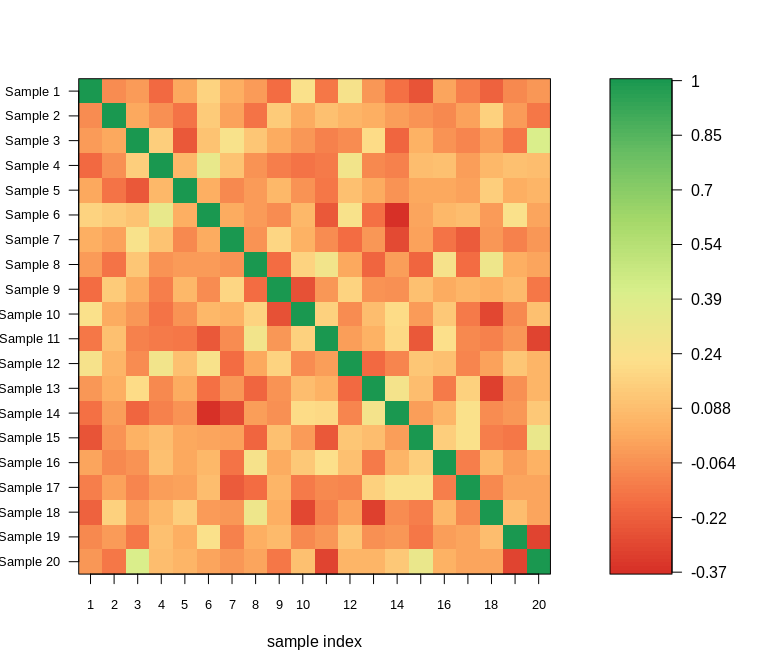Next, we can use the MAD.

## random data
x <- matrix(rnorm(1000), ncol = 10)
## outliers
x[1:20,5] <- x[1:20,5] + 10
title = "MAD: Outlier visible")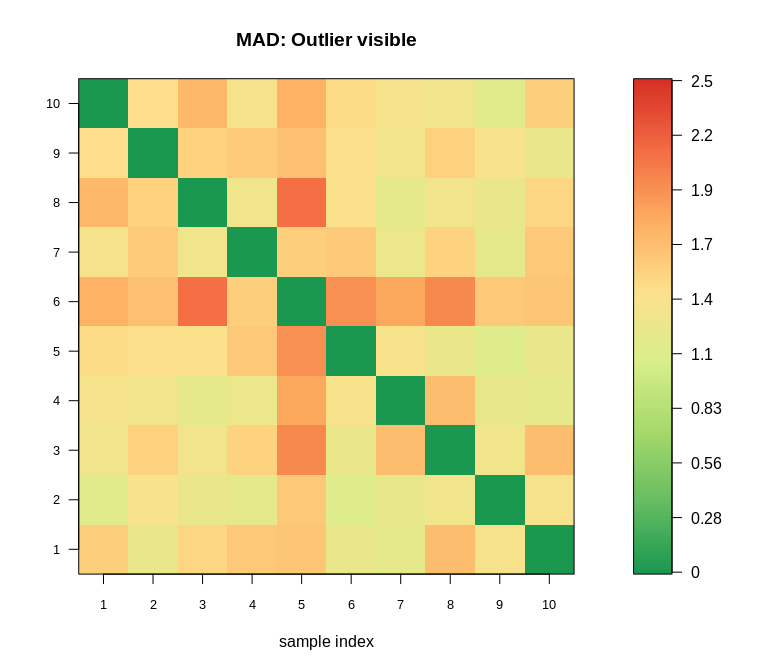## in contrast
corPlot(x, new = TRUE, minCor = -0.5, labels = TRUE,
title = "Correlation: Outlier masked")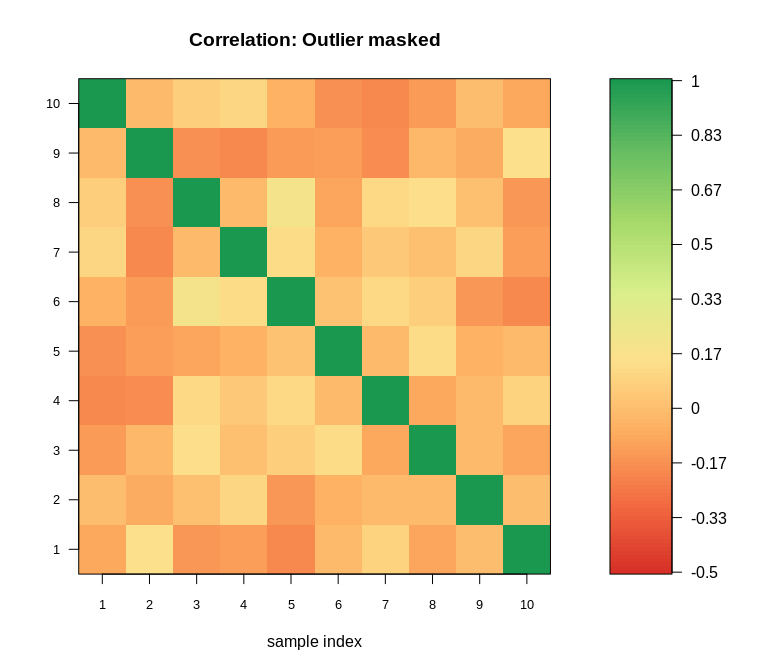### Colors for Heatmaps

Nowadays there are better solutions e.g. provided by Bioconductor package complexHeatmaps. This was my solution to get a better coloring of heatmaps.

## generate some random data
data.plot <- matrix(rnorm(100*50, sd = 1), ncol = 50)
colnames(data.plot) <- paste("patient", 1:50)
rownames(data.plot) <- paste("gene", 1:100)
data.plot[1:70, 1:30] <- data.plot[1:70, 1:30] + 3
data.plot[71:100, 31:50] <- data.plot[71:100, 31:50] - 1.4
data.plot[1:70, 31:50] <- rnorm(1400, sd = 1.2)
data.plot[71:100, 1:30] <- rnorm(900, sd = 1.2)
nrcol <- 128
library(gplots)
##
## Attaching package: 'gplots'
## The following object is masked from 'package:stats':
##
##     lowess
library(RColorBrewer)
myCol <- rev(colorRampPalette(brewer.pal(10, "RdBu"))(nrcol))
heatmap.2(data.plot, col =  myCol, trace = "none", tracecol = "black",
main = "standard colors")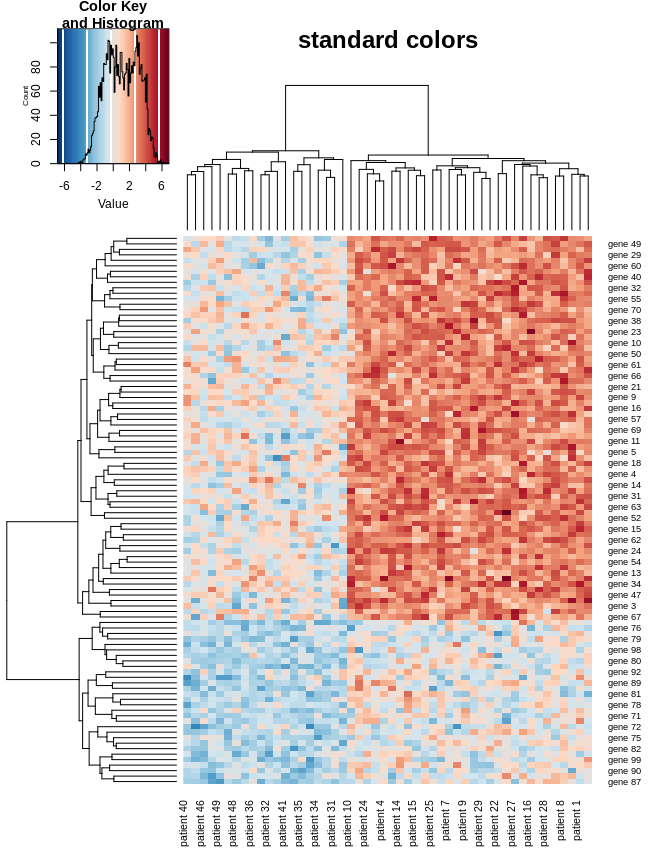farbe <- heatmapCol(data = data.plot, col = myCol,
lim = min(abs(range(data.plot)))-1)
heatmap.2(data.plot, col = farbe, trace = "none", tracecol = "black",
main = "heatmapCol colors")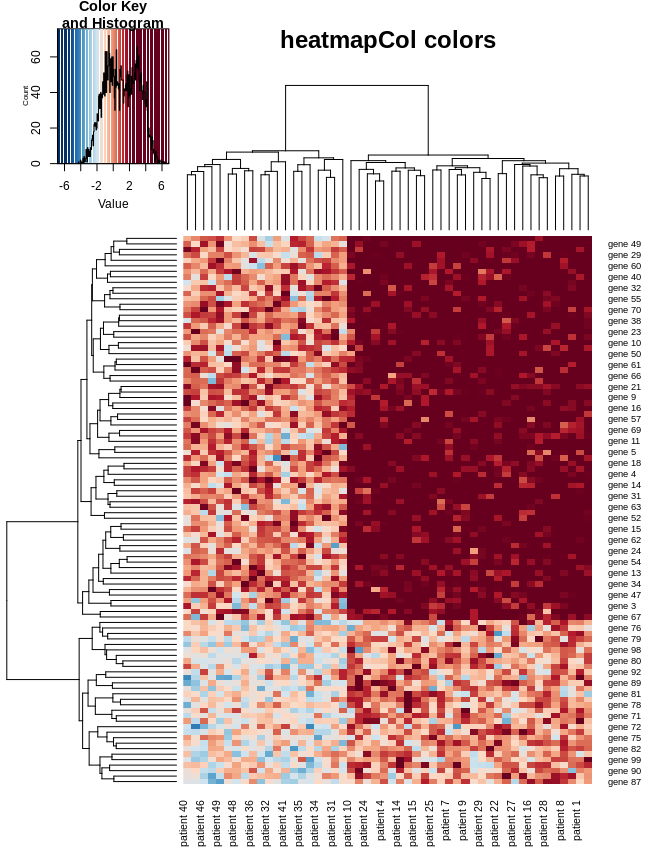## String Alignment

### String Distances

In Bioinformatics the (pairwise and multiple) alignment of strings is an important topic. For this one can use the distance or similarity between strings. The Hamming and the the Levenshtein (edit) distance are implemented in function stringDist.

x <- "GACGGATTATG"
y <- "GATCGGAATAG"
## Hamming distance
stringDist(x, y)
##             GACGGATTATG
## GATCGGAATAG           3
## Levenshtein distance
d <- stringDist(x, y)
d
##             GACGGATTATG
## GATCGGAATAG           3

In case of the Levenshtein (edit) distance, the respective scoring and traceback matrices are attached as attributes to the result.

attr(d, "ScoringMatrix")
##     gap  G A T C G G A A T  A  G
## gap   0  1 2 3 4 5 6 7 8 9 10 11
## G     1  0 1 2 3 4 5 6 7 8  9 10
## A     2  1 0 1 2 3 4 5 6 7  8  9
## C     3  2 1 1 1 2 3 4 5 6  7  8
## G     4  3 2 2 2 1 2 3 4 5  6  7
## G     5  4 3 3 3 2 1 2 3 4  5  6
## A     6  5 4 4 4 3 2 1 2 3  4  5
## T     7  6 5 4 5 4 3 2 2 2  3  4
## T     8  7 6 5 5 5 4 3 3 2  3  4
## A     9  8 7 6 6 6 5 4 3 3  2  3
## T    10  9 8 7 7 7 6 5 4 3  3  3
## G    11 10 9 8 8 7 7 6 5 4  4  3
attr(d, "TraceBackMatrix")
##     gap     G     A     T      C        G      G     A     A      T
## gap "start" "i"   "i"   "i"    "i"      "i"    "i"   "i"   "i"    "i"
## G   "d"     "m"   "i"   "i"    "i"      "m/i"  "m/i" "i"   "i"    "i"
## A   "d"     "d"   "m"   "i"    "i"      "i"    "i"   "m/i" "m/i"  "i"
## C   "d"     "d"   "d"   "mm"   "m"      "i"    "i"   "i"   "i"    "i"
## G   "d"     "d/m" "d"   "d/mm" "d/mm"   "m"    "m/i" "i"   "i"    "i"
## G   "d"     "d/m" "d"   "d/mm" "d/mm"   "d/m"  "m"   "i"   "i"    "i"
## A   "d"     "d"   "d/m" "d/mm" "d/mm"   "d"    "d"   "m"   "m/i"  "i"
## T   "d"     "d"   "d"   "m"    "d/mm/i" "d"    "d"   "d"   "mm"   "m"
## T   "d"     "d"   "d"   "d/m"  "mm"     "d"    "d"   "d"   "d/mm" "m"
## A   "d"     "d"   "d/m" "d"    "d/mm"   "d/mm" "d"   "d/m" "m"    "d"
## T   "d"     "d"   "d"   "d/m"  "d/mm"   "d/mm" "d"   "d"   "d"    "m"
## G   "d"     "d/m" "d"   "d"    "d/mm"   "m"    "d/m" "d"   "d"    "d"
##     A      G
## gap "i"    "i"
## G   "i"    "m/i"
## A   "m/i"  "i"
## C   "i"    "i"
## G   "i"    "m/i"
## G   "i"    "m/i"
## A   "m/i"  "i"
## T   "i"    "i"
## T   "mm/i" "mm/i"
## A   "m"    "i"
## T   "d"    "mm"
## G   "d/mm" "m"

The characters in the trace-back matrix reflect insertion of a gap in string y (d: deletion), match (m), mismatch (mm), and insertion of a gap in string x (i).

### String Similarities

The function stringSim computes the optimal alignment scores for global (Needleman-Wunsch) and local (Smith-Waterman) alignments with constant gap penalties. Scoring and trace-back matrix are again attached as attributes to the results.

## optimal global alignment score
d <- stringSim(x, y)
d
##             GACGGATTATG
## GATCGGAATAG           6
attr(d, "ScoringMatrix")
##     gap  G  A  T  C  G  G  A  A  T   A   G
## gap   0 -1 -2 -3 -4 -5 -6 -7 -8 -9 -10 -11
## G    -1  1  0 -1 -2 -3 -4 -5 -6 -7  -8  -9
## A    -2  0  2  1  0 -1 -2 -3 -4 -5  -6  -7
## C    -3 -1  1  1  2  1  0 -1 -2 -3  -4  -5
## G    -4 -2  0  0  1  3  2  1  0 -1  -2  -3
## G    -5 -3 -1 -1  0  2  4  3  2  1   0  -1
## A    -6 -4 -2 -2 -1  1  3  5  4  3   2   1
## T    -7 -5 -3 -1 -2  0  2  4  4  5   4   3
## T    -8 -6 -4 -2 -2 -1  1  3  3  5   4   3
## A    -9 -7 -5 -3 -3 -2  0  2  4  4   6   5
## T   -10 -8 -6 -4 -4 -3 -1  1  3  5   5   5
## G   -11 -9 -7 -5 -5 -3 -2  0  2  4   4   6
attr(d, "TraceBackMatrix")
##     gap     G     A     T      C      G     G     A     A      T   A
## gap "start" "i"   "i"   "i"    "i"    "i"   "i"   "i"   "i"    "i" "i"
## G   "d"     "m"   "i"   "i"    "i"    "m/i" "m/i" "i"   "i"    "i" "i"
## A   "d"     "d"   "m"   "i"    "i"    "i"   "i"   "m/i" "m/i"  "i" "m/i"
## C   "d"     "d"   "d"   "mm"   "m"    "i"   "i"   "i"   "i"    "i" "i"
## G   "d"     "d/m" "d"   "d/mm" "d"    "m"   "m/i" "i"   "i"    "i" "i"
## G   "d"     "d/m" "d"   "d/mm" "d"    "d/m" "m"   "i"   "i"    "i" "i"
## A   "d"     "d"   "d/m" "d/mm" "d"    "d"   "d"   "m"   "m/i"  "i" "m/i"
## T   "d"     "d"   "d"   "m"    "d/i"  "d"   "d"   "d"   "mm"   "m" "i"
## T   "d"     "d"   "d"   "d/m"  "mm"   "d"   "d"   "d"   "d/mm" "m" "mm/i"
## A   "d"     "d"   "d/m" "d"    "d/mm" "d"   "d"   "d/m" "m"    "d" "m"
## T   "d"     "d"   "d"   "d/m"  "d/mm" "d"   "d"   "d"   "d"    "m" "d"
## G   "d"     "d/m" "d"   "d"    "d/mm" "m"   "d/m" "d"   "d"    "d" "d/mm"
##     G
## gap "i"
## G   "m/i"
## A   "i"
## C   "i"
## G   "m/i"
## G   "m/i"
## A   "i"
## T   "i"
## T   "mm/i"
## A   "i"
## T   "mm"
## G   "m"
## optimal local alignment score
d <- stringSim(x, y, global = FALSE)
d
##             GACGGATTATG
## GATCGGAATAG           6
attr(d, "ScoringMatrix")
##     gap G A T C G G A A T A G
## gap   0 0 0 0 0 0 0 0 0 0 0 0
## G     0 1 0 0 0 1 1 0 0 0 0 1
## A     0 0 2 1 0 0 0 2 1 0 1 0
## C     0 0 1 1 2 1 0 1 1 0 0 0
## G     0 1 0 0 1 3 2 1 0 0 0 1
## G     0 1 0 0 0 2 4 3 2 1 0 1
## A     0 0 2 1 0 1 3 5 4 3 2 1
## T     0 0 1 3 2 1 2 4 4 5 4 3
## T     0 0 0 2 2 1 1 3 3 5 4 3
## A     0 0 1 1 1 1 0 2 4 4 6 5
## T     0 0 0 2 1 0 0 1 3 5 5 5
## G     0 1 0 1 1 2 1 0 2 4 4 6
attr(d, "TraceBackMatrix")
##     gap     G        A           T           C        G
## gap "start" "i"      "i"         "i"         "i"      "i"
## G   "d"     "m"      "i/stop"    "stop"      "stop"   "m"
## A   "d"     "d/stop" "m"         "i"         "i/stop" "d/stop"
## C   "d"     "stop"   "d"         "mm"        "m"      "i"
## G   "d"     "m"      "d/i/stop"  "d/mm/stop" "d"      "m"
## G   "d"     "m"      "mm/i/stop" "stop"      "d/stop" "d/m"
## A   "d"     "d/stop" "m"         "i"         "i/stop" "d"
## T   "d"     "stop"   "d"         "m"         "i"      "i"
## T   "d"     "stop"   "d/stop"    "d/m"       "mm"     "mm/i"
## A   "d"     "stop"   "m"         "d"         "d/mm"   "mm"
## T   "d"     "stop"   "d/stop"    "m"         "i"      "d/mm/i/stop"
## G   "d"     "m"      "i/stop"    "d"         "mm"     "m"
##     G             A          A             T           A        G
## gap "i"           "i"        "i"           "i"         "i"      "i"
## G   "m"           "i/stop"   "stop"        "stop"      "stop"   "m"
## A   "d/mm/stop"   "m"        "m/i"         "i/stop"    "m"      "d/i/stop"
## C   "i/stop"      "d"        "mm"          "mm/i/stop" "d/stop" "mm/stop"
## G   "m/i"         "i"        "d/mm/i/stop" "mm/stop"   "stop"   "m"
## G   "m"           "i"        "i"           "i"         "i/stop" "m"
## A   "d"           "m"        "m/i"         "i"         "m/i"    "i"
## T   "d"           "d"        "mm"          "m"         "i"      "i"
## T   "d"           "d"        "d/mm"        "m"         "mm/i"   "mm/i"
## A   "d/mm/i/stop" "d/m"      "m"           "d"         "m"      "i"
## T   "mm/stop"     "d"        "d"           "m"         "d"      "mm"
## G   "m/i"         "d/i/stop" "d"           "d"         "d/mm"   "m"

The entry stop indicates that the minimum similarity score has been reached.

### Optimal Alignment

Finally, the function traceBack computes an optimal global or local alignment based on a trace back matrix as provided by function stringDist or stringSim.

x <- "GACGGATTATG"
y <- "GATCGGAATAG"
## Levenshtein distance
d <- stringDist(x, y)
## optimal global alignment
traceBack(d)
##    pairwise global alignment
## x' "GA-CGGATTATG"
## y' "GATCGGAATA-G"
## Optimal global alignment score
d <- stringSim(x, y)
## optimal global alignment
traceBack(d)
##    pairwise global alignment
## x' "GA-CGGATTATG"
## y' "GATCGGAATA-G"
## Optimal local alignment score
d <- stringSim(x, y, global = FALSE)
## optimal local alignment
traceBack(d, global = FALSE)
##    pairwise local alignment
## x' "GA-CGGATTA"
## y' "GATCGGAATA"

## sessionInfo

sessionInfo()
## R version 3.6.1 Patched (2019-09-25 r77217)
## Platform: x86_64-pc-linux-gnu (64-bit)
## Running under: Linux Mint 19.2
##
## Matrix products: default
## BLAS:   /usr/lib/x86_64-linux-gnu/libf77blas.so.3.10.3
## LAPACK: /home/kohlm/RTOP/Rbranch/lib/libRlapack.so
##
## locale:
##   LC_CTYPE=de_DE.UTF-8       LC_NUMERIC=C
##   LC_TIME=de_DE.UTF-8        LC_COLLATE=C
##   LC_MONETARY=de_DE.UTF-8    LC_MESSAGES=de_DE.UTF-8
##   LC_PAPER=de_DE.UTF-8       LC_NAME=C
##  LC_MEASUREMENT=de_DE.UTF-8 LC_IDENTIFICATION=C
##
## attached base packages:
##  stats     graphics  grDevices utils     datasets  methods   base
##
## other attached packages:
##  RColorBrewer_1.1-2    gplots_3.0.1.1        exactRankTests_0.8-30
##  Amelia_1.7.5          Rcpp_1.0.2            ggplot2_3.2.1
##  MKmisc_1.6
##
## loaded via a namespace (and not attached):
##   pillar_1.4.2       compiler_3.6.1     DEoptimR_1.0-8
##   bitops_1.0-6       tools_3.6.1        digest_0.6.20
##   evaluate_0.14      tibble_2.1.3       gtable_0.3.0
##  pkgconfig_2.0.2    rlang_0.4.0        yaml_2.2.0
##  xfun_0.9           withr_2.1.2        dplyr_0.8.3
##  stringr_1.4.0      knitr_1.24         caTools_1.17.1.2
##  gtools_3.8.1       grid_3.6.1         tidyselect_0.2.5
##  glue_1.3.1         robustbase_0.93-5  R6_2.4.0
##  foreign_0.8-72     rmarkdown_1.15     gdata_2.18.0
##  limma_3.40.6       purrr_0.3.2        magrittr_1.5
##  scales_1.0.0       htmltools_0.3.6    assertthat_0.2.1
##  colorspace_1.4-1   labeling_0.3       KernSmooth_2.23-15
##  stringi_1.4.3      lazyeval_0.2.2     munsell_0.5.0
##  crayon_1.3.4